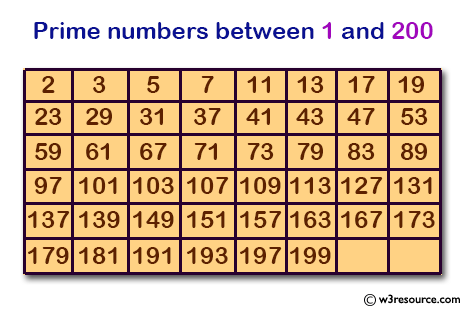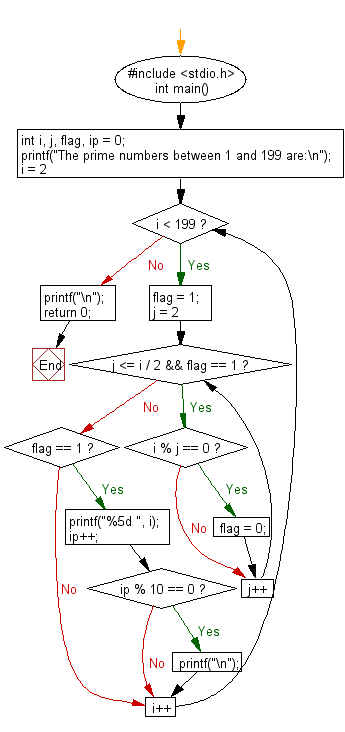﻿ C Program: Prints out the prime numbers between 1 and 200 - w3resource

# C Exercises: Prints out the prime numbers between 1 and 200

## C Basic Declarations and Expressions: Exercise-65 with Solution

Write a C program that generates 50 random numbers between -0.5 and 0.5 and writes them to the file rand.dat. The first line of ran.dat contains the number of random numbers, while the next 50 lines contain 50 random numbers.

Expected output:
The prime numbers between 1 and 199 are:
2 3 5 7 11 13 17 19 23 29
31 37 41 43 47 53 59 61 67 71
73 79 83 89 97 101 103 107 109 113
127 131 137 139 149 151 157 163 167 173
179 181 191 193 197

Pictorial Presentation:Sample Solution:

C Code:

``````#include <stdio.h>
int main() {
int i, j, flag, ip = 0;

// Print a message indicating the purpose of the program
printf("The prime numbers between 1 and 199 are:\n");

// Loop to check prime numbers from 2 to 198
for (i = 2; i < 199; i++) {
flag = 1;

// Loop to check if i is divisible by any number other than 1 and itself
for (j = 2; j <= i / 2 && flag == 1; j++) {
if (i % j == 0) {
flag = 0;
}
}

// If i is prime, print it
if (flag == 1) {
printf("%5d ", i);
ip++;

// Print a newline character after every 10 prime numbers
if (ip % 10 == 0) {
printf("\n");
}
}
}

printf("\n");

return 0;
}
``````

Sample Output:

```The prime numbers between 1 and 199 are:
2     3     5     7    11    13    17    19    23    29
31    37    41    43    47    53    59    61    67    71
73    79    83    89    97   101   103   107   109   113
127   131   137   139   149   151   157   163   167   173
179   181   191   193   197
```

Flowchart:C programming Code Editor:

What is the difficulty level of this exercise?

Test your Programming skills with w3resource's quiz.

﻿